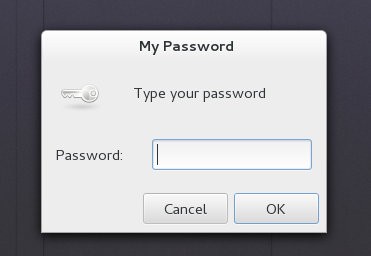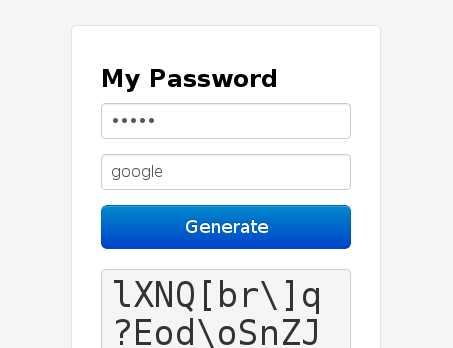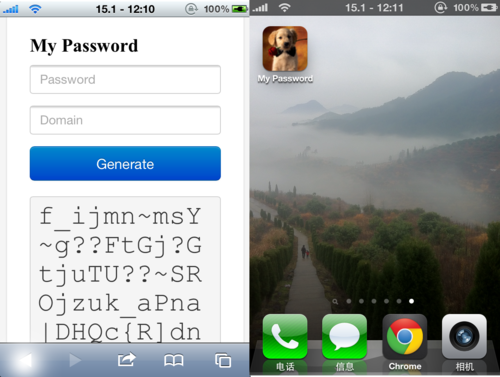I'm 赵一开, a developer.

Get in touch:## 我是如何管理密码的

### 刚开始的想法是

• 自己牢记一个通用的“密码”，只用于生成密码，记为p。
• 将p做10次sha512sum。
• 再将p做10次sha512sum。
• 取p的一个字串为该网站密码。

``````  for i in xrange(10):
passwd = sha512(passwd).hexdigest()
ret = passwd + domain
for i in xrange(10):
ret = sha512(ret).hexdigest()

``````

### 为了减小暴力破解的可能

``````splitLine = lambda s, n: [s[n*i:n*i+n] for i in xrange(len(s)/n)]
b = bin(int(s, 16)).partition('b')
chs = splitLine(b, 6)
ret = [chr(int(ch, 2) + 63) for ch in chs]
return ''.join(ret)

``````

### 当心身后的人

``````> python mypasswd.py google
lXNQ[br\]q
?Eod\oSnZJ
N^_t[QQGoY
CRxFlxMdUS
muMbQiJyV_
kftVs{`Fk|
_kmKionj@i
qyXEf|Jz_e

``````

### Easily Access

#### 命令行

``````passwd = getpass('Password: ')
domain = ' '.join(sys.argv[1:])
ret = '\n'.join(generate(passwd, domain))
print ret

``````

#### GUI

``````import subprocess
passwd = subprocess.check_output(
domain = subprocess.check_output(
ret = '\n'.join(generate(passwd, domain))
fname = os.path.join(gettempdir(), 'my_passwd')
with open(fname, 'w') as f:
f.write(ret)
try:
subprocess.check_output(
['zenity', '--width=50', '--height=330', '--text-info', \
except subprocess.CalledProcessError:
pass
os.remove(fname)

``````#### 网页端